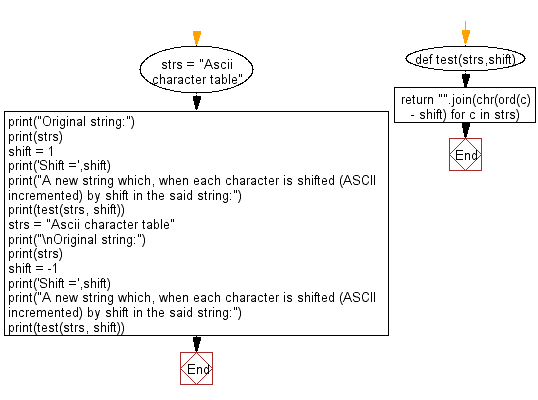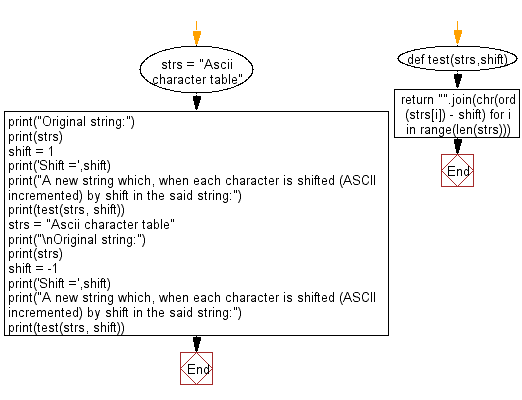﻿ Python: Find a string which, when each character is shifted (ASCII incremented) by shift - w3resource# Python: Find a string which, when each character is shifted (ASCII incremented) by shift

## Python Programming Puzzles: Exercise-67 with Solution

Write a Python program to find a string which, when each character is shifted (ASCII incremented) by shift, gives the result.

ASCII table -

```Input:
Ascii character table
Shift = 1
Output:
@rbhhbg`q`bsdqs`akd

Input:
Ascii character table
Shift = -1
Output:
Btdjj!dibsbdufs!ubcmf
```

Sample Solution-1:

Python Code:

``````#License: https://bit.ly/3oLErEI

def test(strs,shift):
return "".join(chr(ord(c) - shift) for c in strs)

strs = "Ascii character table"
print("Original string:")
print(strs)
shift = 1
print('Shift =',shift)
print("A new string which, when each character is shifted (ASCII incremented) by shift in the said string:")
print(test(strs, shift))
strs = "Ascii character table"
print("\nOriginal string:")
print(strs)
shift = -1
print('Shift =',shift)
print("A new string which, when each character is shifted (ASCII incremented) by shift in the said string:")
print(test(strs, shift))
``````

Sample Output:

```Original string:
Ascii character table
Shift = 1
A new string which, when each character is shifted (ASCII incremented) by shift in the said string:
@rbhhbg`q`bsdqs`akd

Original string:
Ascii character table
Shift = -1
A new string which, when each character is shifted (ASCII incremented) by shift in the said string:
Btdjj!dibsbdufs!ubcmf
```

Flowchart:## Visualize Python code execution:

The following tool visualize what the computer is doing step-by-step as it executes the said program:

Sample Solution-2:

Python Code:

``````#License: https://bit.ly/3oLErEI

def test(strs,shift):
return "".join(chr(ord(strs[i]) - shift) for i in range(len(strs)))

strs = "Ascii character table"
print("Original string:")
print(strs)
shift = 1
print('Shift =',shift)
print("A new string which, when each character is shifted (ASCII incremented) by shift in the said string:")
print(test(strs, shift))
strs = "Ascii character table"
print("\nOriginal string:")
print(strs)
shift = -1
print('Shift =',shift)
print("A new string which, when each character is shifted (ASCII incremented) by shift in the said string:")
print(test(strs, shift))
``````

Sample Output:

```Original string:
Ascii character table
Shift = 1
A new string which, when each character is shifted (ASCII incremented) by shift in the said string:
@rbhhbg`q`bsdqs`akd

Original string:
Ascii character table
Shift = -1
A new string which, when each character is shifted (ASCII incremented) by shift in the said string:
Btdjj!dibsbdufs!ubcmf
```

Flowchart:## Visualize Python code execution:

The following tool visualize what the computer is doing step-by-step as it executes the said program:

Python Code Editor :

Have another way to solve this solution? Contribute your code (and comments) through Disqus.

What is the difficulty level of this exercise?

Test your Programming skills with w3resource's quiz.

﻿

## Python: Tips of the Day

Clamps num within the inclusive range specified by the boundary values x and y:

Example:

```def tips_clamp_num(num,x,y):
return max(min(num, max(x, y)), min(x, y))
print(tips_clamp_num(2, 4, 6))
print(tips_clamp_num(1, -1, -6))
```

Output:

```4
-1
```